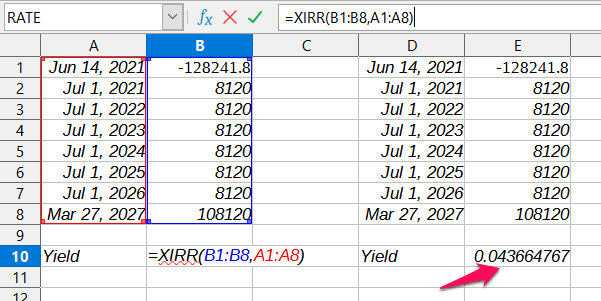# NCD YTM calculation

Tenure|Price/unit|YTM|Coupon|Frequency|Face Value|
71 months|1,276.75|4.16%|8.12%|Yearly|1,000|

This info is for REC Ltd Bond as shown in Coin… Here I tried to calculate the YTM myself but a different value is coming up instead of 4.16%. Please guide if there’s something wrong with the calculation or something else is missing?

Calculation -
If 100 qty is bought then buy price will be 127675, rate of interest is 8.12% so annual interest will be 8120 as face value is 1000.
Total invested years are 5.916. So total interest for all those years will be 8120*5.916 = 48037.92.
Now, to get the net profit if bond is held till maturity we’ll subtract the difference in buy price and face value, 127675-100000 = 27675 from the total interest. net profit = 48037.92-27675 = 20362.92.
YTM = (20362.92/127675)*100 = 15.949 now we divide this with no. of years 15.949/5.916 = 2.69%

I dunno if this is even the right way to calculate this but I saw this method in varsity, Please help!

@neha1101

2 Likes

Any help!

From the information that I could find, this bond (INE020B07GH7) pays interest once a year on 1st July and the last interest payment will be on the maturity date along with the principal amount. If we take that into account, then 7 payments remain (for 2021, 2022, 2023, 2024, 2025, 2026, 2027). I found an article, which suggests using the XIRR function in Excel to calculate YTM. Upon doing that, my results overshoot the interest rate shown on the website (4.36% instead of 4.07%) -The market price for Bond have changed as follows -

From what I am able to understand, the mismatch is happening because of not taking into account accrued interest (This is explained in an example in this video). Roughly, if I add around Rs.1700 into the acquisition amount, then the YTM matches with the website aka 4.07% but I am not able to understand how that amount is arrived at. If anyone knows about it, do share.

3 Likes

Hi,

From the computations shared, I think the terminal year payment (i.e., payment made on 27-Mar-2027) should be ~ Rs. 105,984 (100,000 + 5,984), since the period between the last and penultimate payouts is ~ 269 days [100,000 * 8.12% * 269 / 365 = 5,984].

If this change were to be considered, the YTM matches with the one mentioned in the website.

2 Likes

@Prayag Thanks for the explanation. Could you have a look at this INE721A07HL2 BOND 9.71% PA SRN S-V 15-Jul-2021

As this bond is going to mature next month and even its last price is 1011. If some1 buys this bond right now will he be getting full interest on the amount invested on 15 July 2021? even though he invested just a month ago? cuz if so then by your xirr method thats a 170% YTM

This bond pays interest on a monthly basis (INE721A07HL2). This can also be verified from the document available on their website -

This bond has an effective coupon rate of 10.94% for individuals. That would mean a monthly payment of Rs. 9.11 ( (1000 * 0.1094) / 12) ) and around Rs. 4.19 for the remaining 14 days ( ((1000 * 0.1094) / 365) * 14 ) on maturity along with the face value. Using this, the YTM comes out to be 2.7% -

The reason the yield comes out to be that number is because XIRR function returns effective annual yield (Refer to Quant Bonds - Between Coupon Dates).

1 Like

@Prayag Thanks a ton for the clarification. Just 1 last question. This is the ISIN of the bond INE721A07OB9. Here the XIRR came 8.658%, is it correct?

Also the absolute return for this bond will be 8.43%? I calculated this as follows for 100qty -
760(per month interest)*29(no. of interest payout months) = 22040 - 2000(the loss when sold at face value as last price of bond is 1020) = 20040 / 102000(buy price) = 0.1964 / 2.33 (no. of years) = 0.0843 * 100 = 8.43% . Is this calculation for absolute return correct ?

ty in adv!

Using the XIRR function, the return for that bond (INE721A07OB9) indeed come out to be around your estimate (8.64%) -

The actual YTM would be a little lower because the XIRR function assumes that all interest payouts were re-invested back into the bond (which might not be the case). As per the IndiMoney website, this bond has a YTM of 8.20%

For absolute return, I did the calculation by adding all the interest payments and the face value of the bond ( 100000 + 29 * 760 = 122040 ) and then subtracting the initial investment from it (122040 - 102000 = 20040) and finally dividing it by the initial investment ( 20040 / 102000 = 0.196470588 * 100 = 19.64%)

2 Likes

@Prayag 19.64 needs to b divided by 2.33 to get per year % ?

For that, use the CAGR (Compounded Annual Growth Rate) formula -( ( 122040 / 102000 ) ^ ( 1 / 2.38535361 ) ) - 1 = 0.0780985991 * 100 = 7.8%

** - 2 years 4 months 19 days is 2.38535361 years

1 Like